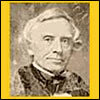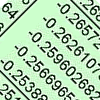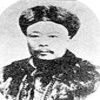#### You may also like### History of Morse

This short article gives an outline of the origins of Morse code and its inventor and how the frequency of letters is reflected in the code they were given.### Odd One Out

In this short problem, can you deduce the likely location of the odd ones out in six sets of random numbers?### Very Old Man

Is the age of this very old man statistically believable?

# The Wrong Stats

##### Age 16 to 18 Challenge Level:

Here are some statistical and probabilistic statements. However reasonable they might sound, they CANNOT be completely true, or might even be completely false. They are, at best, an approximation, although the approximation might be very good. Why?

1. I toss a coin. The chance of landing heads is $1$ minus the chance of landing tails.
2. The energy of a randomly selected atom in a box is normally distributed with some mean and variance.
3. My pdf is a semi-circle of radius 1.
4. For any piece of uranium, half of the mass will decay every time the half-life elapses.
5. I record the time of day at which an event occurs, with the knowledge that the event is just as likely to occur at any time as any other. The time measured will be a $U(0,1)$ random variable.
6. An influenza pandemic is just as likely to occur one year as any other.
7. The arithmetic mean of the number of children per family in a certain population is $a$. Therefore, the arithmetic mean of the number of siblings each child has is $a-1$.
8. The expected age of death of a randomly selected $40$ year old is the same as the expected age of death of a randomly selected $35$ year old.

Can you invent your own statistical statements which sound plausible but must be false?